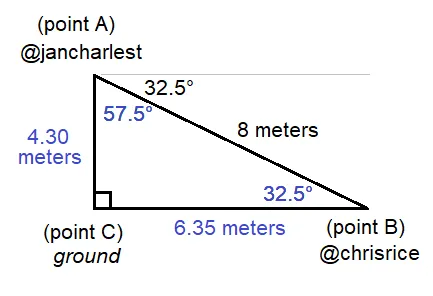RE: RE: Math mini-contest problem for Day 7 😎
You are viewing a single comment's thread from:

RE: Math mini-contest problem for Day 7 😎

# 4.30 meters 🎯

The problem is under trigonometry.

Let A be the point where the tip of @jancharlest's laser gun is at. Let B be the point where the target @chrisrice is. Let C be the point of intersection of the vertical line where @jancharlest is at and the horizontal ground line, which has an angle of 90°.

Since the aim of @jancharlest is basically a laser point on the target, the target @chrisrice will be represented as a point. @jancharlest is aiming 32.5° downwards, which is the same angle as @chrisrice looking up to @jancharlest's laser gun tip.

We now have a logical triangle ABC. The given data are AC = 8 meters, ACB = 90°, and ABC = 32.5%. By derivation, we get CAB = 57.5% by deducting the two known angles from 180° which is the sum of all angles of a triangle. Since all angles are known, we can use either the Law of Sines, Law of Cosines, or Law of Tangents. For this solution, we use the Law of Sines because it is the most simple among the three formulas.

Law of Sines: a / sin (A) = b / sin (B) = c / sin (C)
For the original problem, we will use b / sin (B) = c / sin (C)

In this solution, [side b] is AC (which is the unknown in this equation) and angle B is CBA or 32.5°, while [side c] is 8 meters and angle C is ACB or 90°.

AC / sin (32.5°) = 8 meters / sin (90°)
AC / 0.5373 = 8 meters / 1
AC = 0.5373 * 8 meters
AC = 4.2984 meters

Therefore, the distance between the ground and @jancharlest's laser gun tip, or basically the height, is approximately 4.30 meters. We can have multiple solutions for the problem, but the answer shall be the same.

To help understand the big picture, here is an image I made using Microsoft Paint:## Winner: none 🤯

I should post another Math problem soon to complete the 7 days with 7 winning answers.